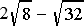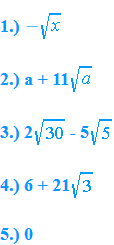Like square roots are square roots with the same radicands.  Like square roots can be added or subtracted in the same manner as like terms are added and subtracted.

Example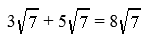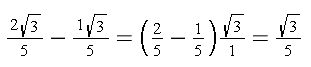Unlike square roots have different radicands.  It is sometimes possible to change unlike square roots into like square roots by simplifying the radicals into an expression.  After simplifying, the terms containing the same radicand can be combined.

Example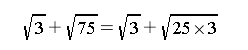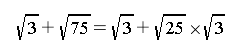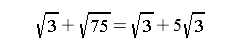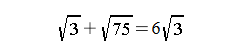Practice

1)  Simplify the square roots: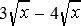2)  Simplify: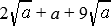3)  Simplify: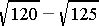4)  Simplify: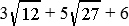5)  Simplify: